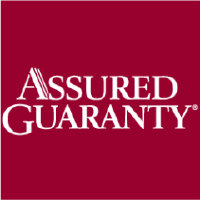# Assured Guaranty Ltd (NYSE:AGO)

57.88 USD +3.22 USD ( +5.89% )Assured Guaranty Ltd
NYSE:AGO

## DCF Value

Estimated DCF Value of oneAGO stock under the base case scenario is 109.15 USD. Compared to the current market price of 57.88 USD, the stock is Undervalued by 47%.

Estimated DCF Value of one NYSE:AGO stock is 109.15 USD. Compared to the current market price of 57.88 USD, the stock is Undervalued by 47% .

DCF valuation is one of two methods of placing a monetary value on a company; the other is Relative Valuation method. We use a combination of these two methods to calculate the Intrinsic Value of stock as accurately as possible.

Discount Rate
8.42%
Terminal Growth
0%
Growth Period
5 Years
Discount Rate
8.42%
Terminal Growth
0%
Growth Period
5 Years

You can change any inputs, such as future revenue, using Present Value Calculation block.

DCF Valuation FAQ:
AGO DCF Value
Base Case
109.15 USD
Undervaluation 47%
DCF Value
PriceAssured Guaranty Ltd Competitors:
DCF Valuation
8766
Tokio Marine Holdings Inc
STFC
State Auto Financial Corp
PLMR
Palomar Holdings Inc
AXS
AXIS Capital Holdings Ltd

## DCF Value Calculation

### Capital Structure From Present Value to DCF ValuePresent Value 7.4B USD Equity Value 7.4B USD / Shares Outstanding 67.4M AGO DCF Value 109.15 USD
Undervalued by 47%

To view the process of calculating the Present Value of Assured Guaranty Ltd' future free cash flow, see the Present Value Calculation block.

## Present Value Calculation

### Discounted Cash Flow Model Present Value of Free Cash FlowRotate your device for better experience.
Currency: USD

To view the process of deriving the DCF Value of one share from the estimated Present Value, see the DCF Value Calculation block.

## Sensitivity Analysis

### Sensitivity Analysis DCF Value Sensitivity AnalysisAnalyze the possible values of AGO stock DCF Value given various model inputs such as Revenue Growth, Operating Margin, and Discount Rate.

Similar Stocks

## AGO Stock DCF Valuation FAQ

What is the DCF value of one AGO stock?

Estimated DCF Value of oneAGO stock under the base case scenario is 109.15 USD. Compared to the current market price of 57.88 USD, the stock is Undervalued by 47%.

The true DCF Value lies somewhere between the worst-case and best-case scenario values. This is because the future is not predetermined, and the stock's DCF Value is based almost entirely on the future of the company. Knowing the full range of possible stock intrinsic values gives a complete picture of the investment risks and opportunities.

How was the DCF Value calculated?

1. Present Value Calculation. Using the DCF Operating Model we projectAssured Guaranty Ltd's future free cash flow and discount it at a selected discount rate to calculate its Present Value (7.4B USD).

2. DCF Value Calculation. We use the company's capital structure to calculate the total Equity Value based on the previously computed Present Value of the free cash flow. Dividing the Equity Value by the number of shares outstanding gives us the DCF Value of 109.15 USD per oneAGO share.